trustle > EngliSea > Corona Safe Schooling > mathematics > Quick Math Index > 20 Algebra

The study of mathematical symbols and the rules for manipulating these symbols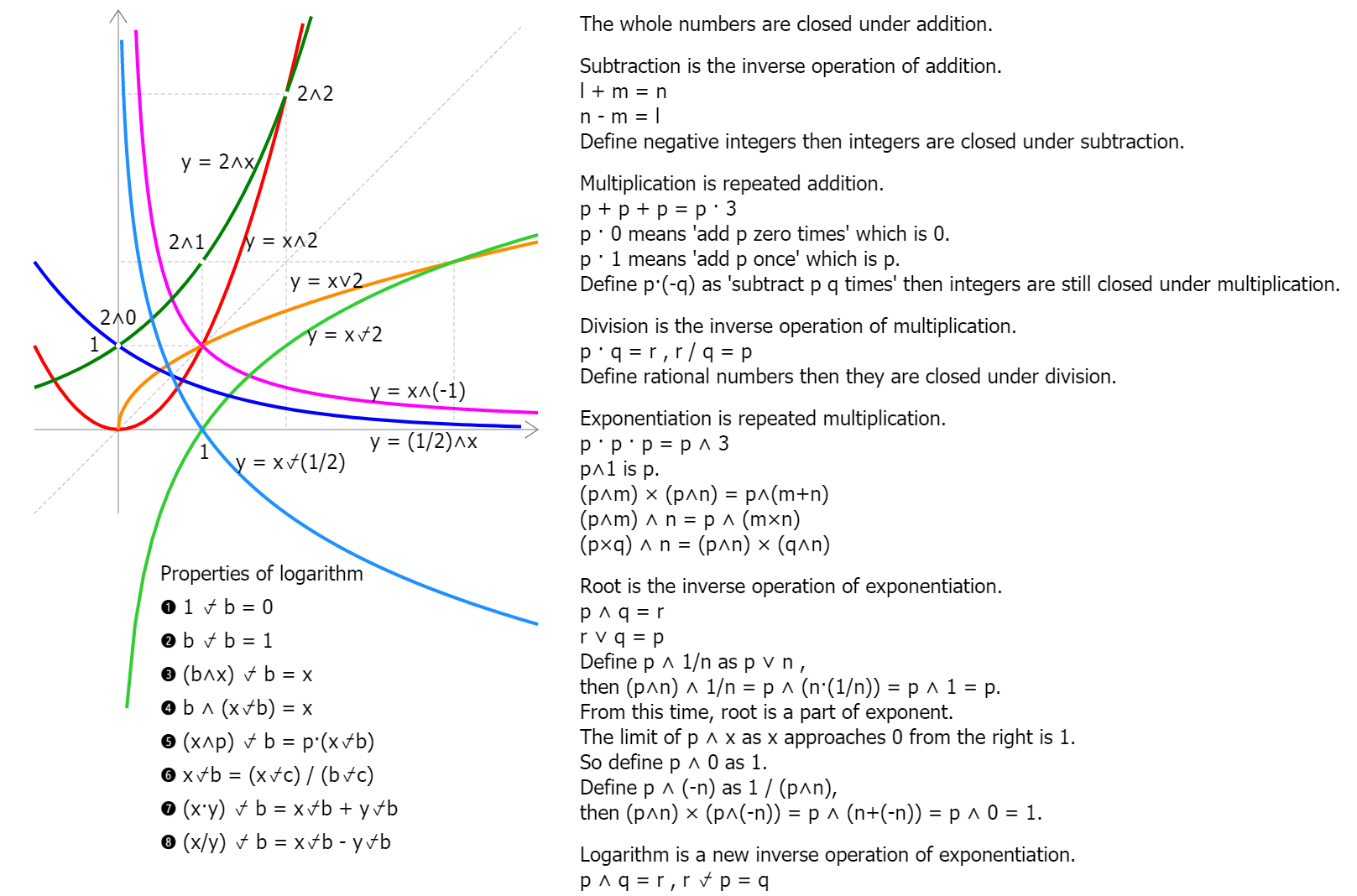Expansion of Numbers and Operations
3699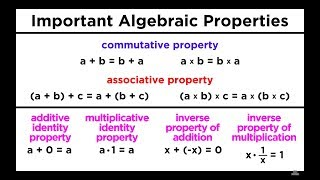02 Basic Number Properties for Algebra
Basic Number Properties for Algebra
4987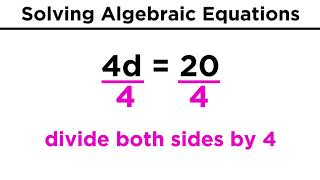03 Algebraic Equations and Their Solutions
Algebraic Equations and Their Solutions
4988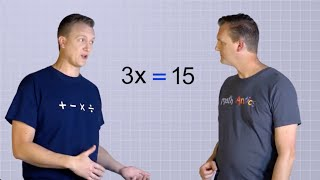03 Solving Basic Equations Part 2
Algebra Basics: Solving Basic Equations Part 2 - Math Antics
5128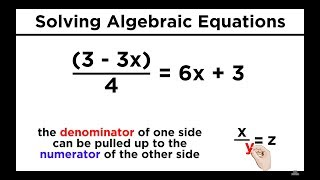04 Algebraic Equations With Variables on Both Sides
Algebraic Equations With Variables on Both Sides
4989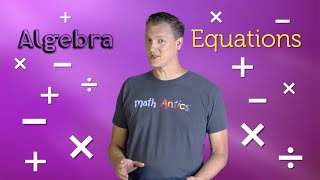04 Solving 2-Step Equations
Algebra Basics: Solving 2-Step Equations - Math Antics
5129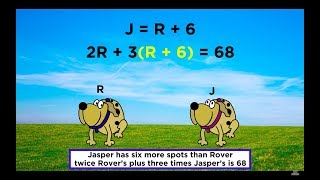05 Algebraic Word Problems
Algebraic Word Problems
4990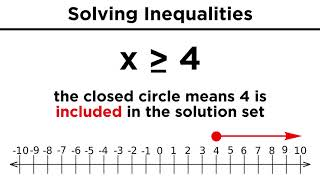06 Solving Algebraic Inequalities
Solving Algebraic Inequalities
4991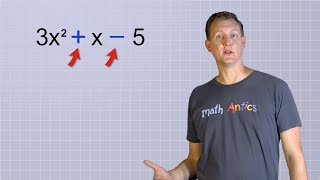06 What Are Polynomials?
Algebra Basics: What Are Polynomials? - Math Antics
5130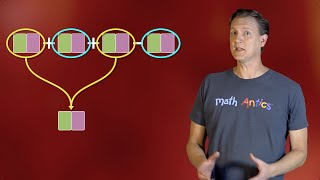07 Simplifying Polynomials
Algebra Basics: Simplifying Polynomials - Math Antics
5131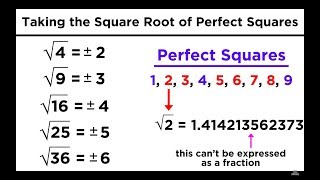07 Square Roots, Cube Roots, and Other Roots
Square Roots, Cube Roots, and Other Roots
4992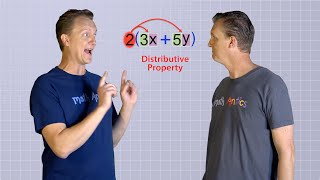08 The Distributive Property
Algebra Basics: The Distributive Property - Math Antics
5132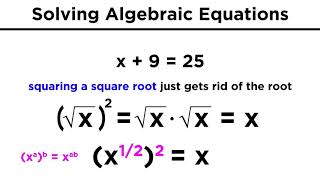09 Solving Algebraic Equations With Roots and Exponents
Solving Algebraic Equations With Roots and Exponents
4994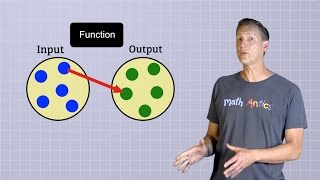10 Functions
Algebra Basics: What Are Functions? - Math Antics
5074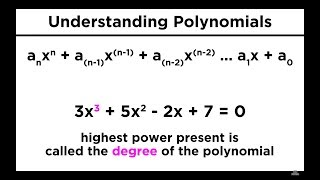10 Introduction to Polynomials
Introduction to Polynomials
4995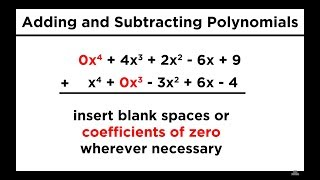11 Adding and Subtracting Polynomials
4996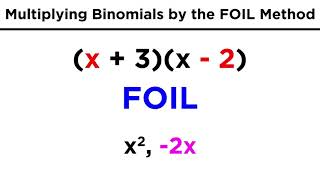12 Multiplying Binomials by the FOIL Method
Multiplying Binomials by the FOIL Method
4997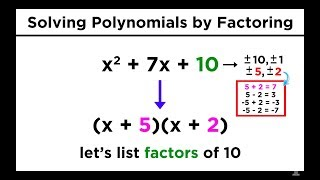13 Solving Quadratics by Factoring
4998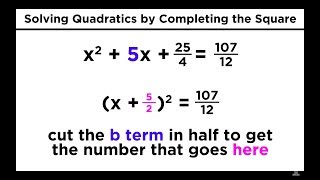14 Solving Quadratics by Completing the Square
Solving Quadratics by Completing the Square
5082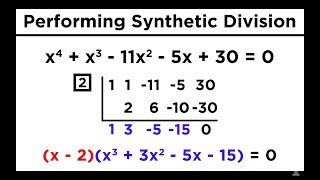16 Solving Higher Degree Polynomials by Synthetic Division and the Rational Roots Test
Solving Higher Degree Polynomials by Synthetic Division and the Rational Roots Test
5084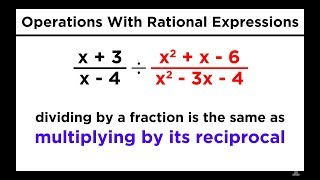17 Manipulating Rational Expressions: Simplification and Operations
Manipulating Rational Expressions: Simplification and Operations
5085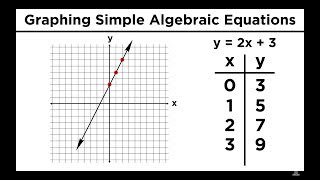18 Graphing in Algebra: Ordered Pairs and the Coordinate Plane
Graphing in Algebra: Ordered Pairs and the Coordinate Plane
5086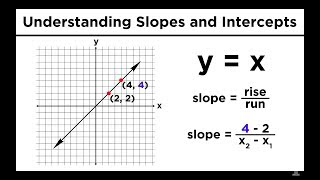19 Graphing Lines in Algebra: Understanding Slopes and Y-Intercepts
Graphing Lines in Algebra: Understanding Slopes and Y-Intercepts
5087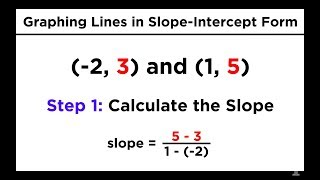20 Graphing Lines in Slope-Intercept Form (y = mx + b)
Graphing Lines in Slope-Intercept Form (y = mx + b)
5088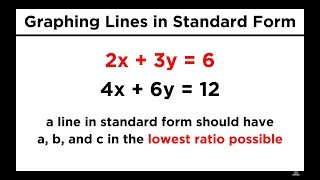21 Graphing Lines in Standard Form (ax + by = c)
Graphing Lines in Standard Form (ax + by = c)
5089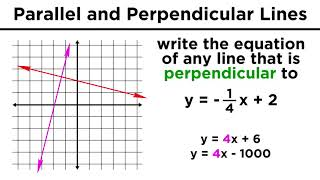22 Graphing Parallel and Perpendicular Lines
Graphing Parallel and Perpendicular Lines
5090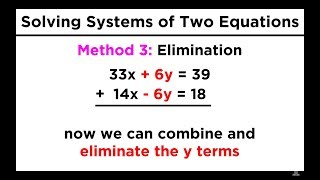23 Solving Systems of Two Equations and Two Unknowns: Graphing, Substitution, and Elimination
Solving Systems of Two Equations and Two Unknowns: Graphing, Substitution, and Elimination
5091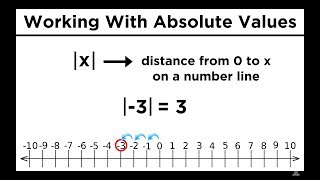24 Absolute Values: Defining, Calculating, and Graphing
Absolute Values: Defining, Calculating, and Graphing
5261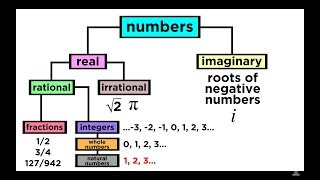25 What are the Types of Numbers?
Real vs. Imaginary, Rational vs. Irrational
5262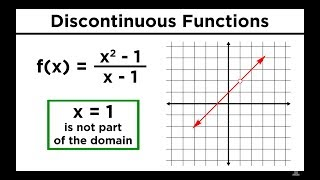30 Continuous, Discontinuous, and Piecewise Functions
Continuous, Discontinuous, and Piecewise Functions
5263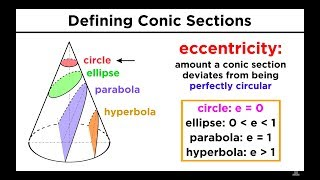33 Graphing Conic Sections Part 1: Circles
Graphing Conic Sections Part 1: Circles
5264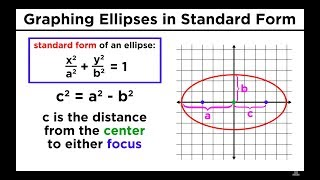34 Graphing Conic Sections Part 2: Ellipses
Graphing Conic Sections Part 2: Ellipses
5265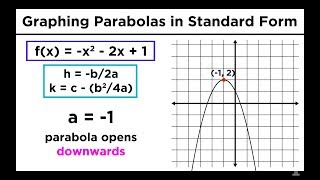35 Graphing Conic Sections Part 3: Parabolas in Standard Form
Graphing Conic Sections Part 3: Parabolas in Standard Form
5266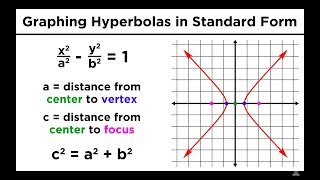36 Graphing Conic Sections Part 4: Hyperbolas
Graphing Conic Sections Part 4: Hyperbolas
5267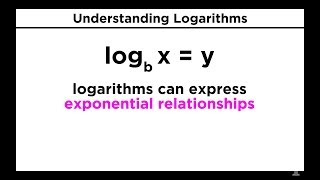41 Logarithms Part 1
When we say log base b of x equals y, we are saying b to the y equals x. With logs, the base of the log raised to the power of what's on the other side of the equal sign will equal the number that the log is operating on.
5040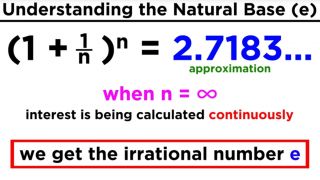42 Logarithms Part 2
Base Ten Logs, Natural Logs, and the Change-Of-Base Property
5041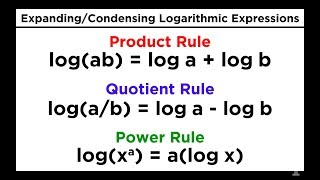43 Logarithms Part 3
Logarithms Part 3: Properties of Logs, Expanding Logarithmic Expressions
5092-